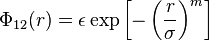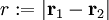# Difference between revisions of "Generalized exponential model"

The Generalized exponential model (or GEM-m potential) is given by (Eq. x in ):$\Phi_{12}(r) = \epsilon \exp \left[ -\left( \frac{r}{\sigma} \right)^m \right]$

where

•$r := |\mathbf{r}_1 - \mathbf{r}_2|$
•$\Phi_{12}(r)$ is the intermolecular pair potential between two particles or sites

## m=1

Also known as the EXP model .

## m=4

2-dimensions .